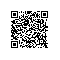# 九度题目1333：考研海报

sun是万千考研学子中的一员，他每天过着三点一线的生活。

sun提出了一个问题：公告栏上还剩多少空白区域是没被考研海报张贴过的？

3

2

0 0 1 2

0 0 2 1

10

2

0 0 1 1

0 0 3 3

6

913 2

0 0 1 1

2 2 3 3

AC代码：

#include<stdio.h>
#include<string.h>
int a,b,f;
int main()
{
int i,j,n,m,sum,x1,y1,x2,y2,p,k,v,u;
while(scanf("%d %d",&n,&m)!=EOF)
{
memset(a,0,sizeof(a));
memset(b,0,sizeof(b));
memset(f,0,sizeof(f));
for(i=0;i<m;i++)
{
scanf("%d%d%d%d",&x1,&y1,&x2,&y2);
if(x1<n&&y1<n&&x2<=n&&y2<=n&&x1>=0&&y1>=0&&x2>0&&y2>0)//由于坐标会超出范围，所以要确定坐标范围
{
for(p=x1;p<x2;p++)//左上方x坐标
for(k=y1;k<y2;k++)//左上方y坐标
{
a[p][k]=1;//左上方x坐标和左上方y坐标
b[p+1][k+1]=1;//右下方x坐标和右下方y坐标
f[p][k+1]=1;//防止图2得情况发生，要加一个判定坐标
}
}
else
{
//将超出范围的坐标缩小到所给范围内在计算
if(x2>n||y2>n)
{
while(!(x2<=n&&y2<=n))
{
if(x2>n)
x2--;
if(y2>n)
y2--;
}

}

if(x1<0||y1<0)
{
while(!(x1>=0&&y1>=0))
{
if(x1<0)
x1++;
if(y1<0)
y1++;
}
}

for(p=x1;p<x2;p++)//左上方x坐标
for(k=y1;k<y2;k++)//左上方y坐标
{
a[p][k]=1;//左上方x坐标和左上方y坐标
b[p+1][k+1]=1;//右下方x坐标和右下方y坐标
f[p][k+1]=1;//防止图2得情况发生，要加一个判定坐标
}
}
}
int sum=0;
for(i=0;i<n;i++)
for(j=0;j<n;j++)
{
//找到没有被占位的，记录
if(a[i][j]==0&&b[i+1][j+1]==0&&f[i][j+1]==0)
sum++;
}
printf("%d\n",sum);
}
return 0;
}使用钉钉扫一扫加入圈子
+ 订阅